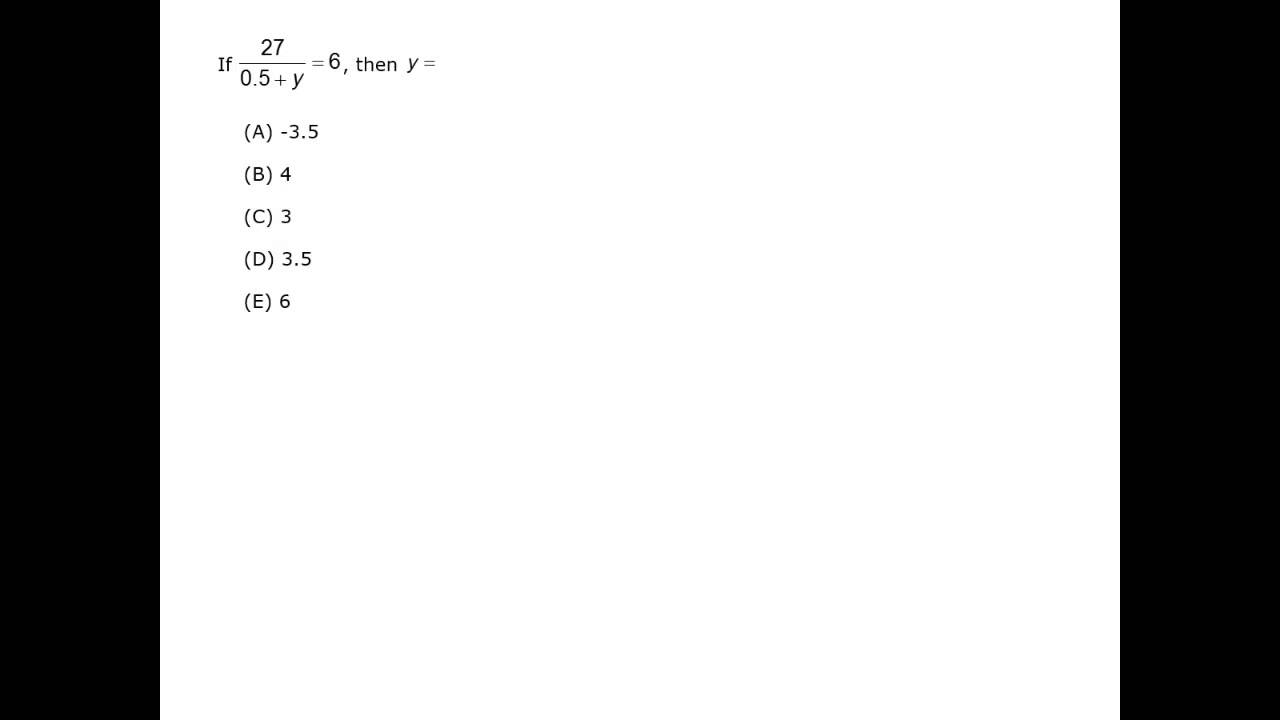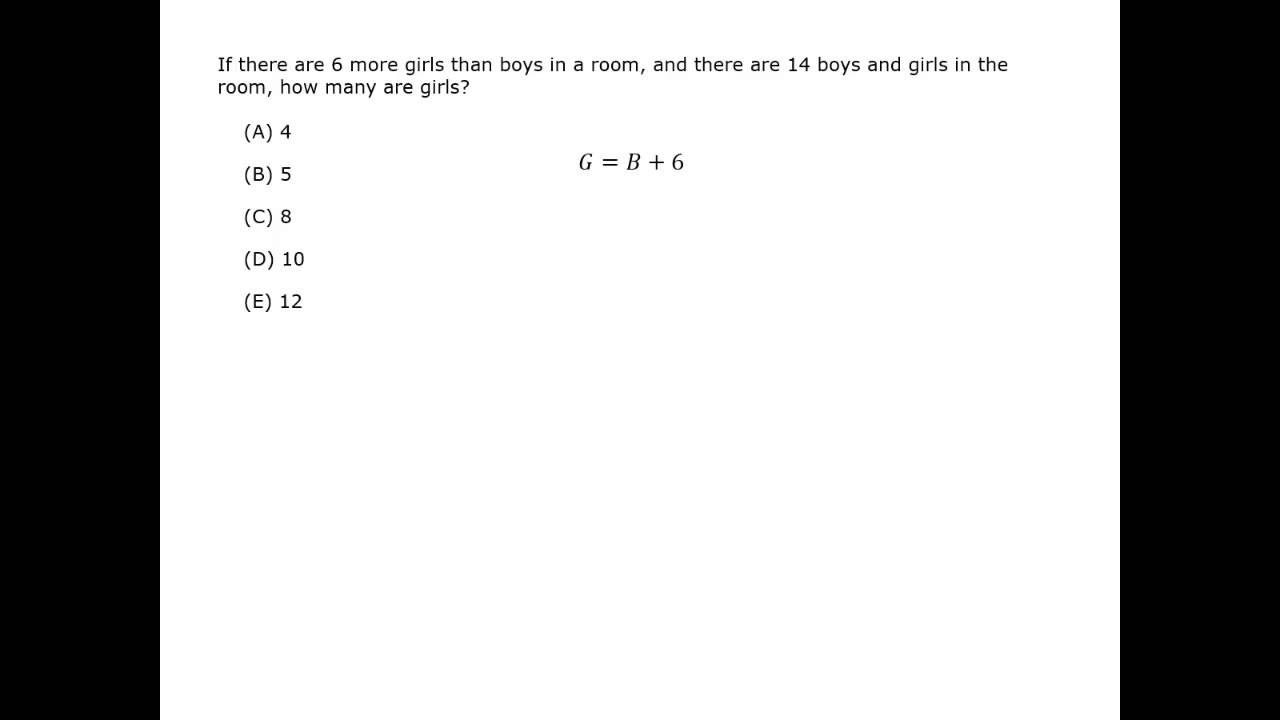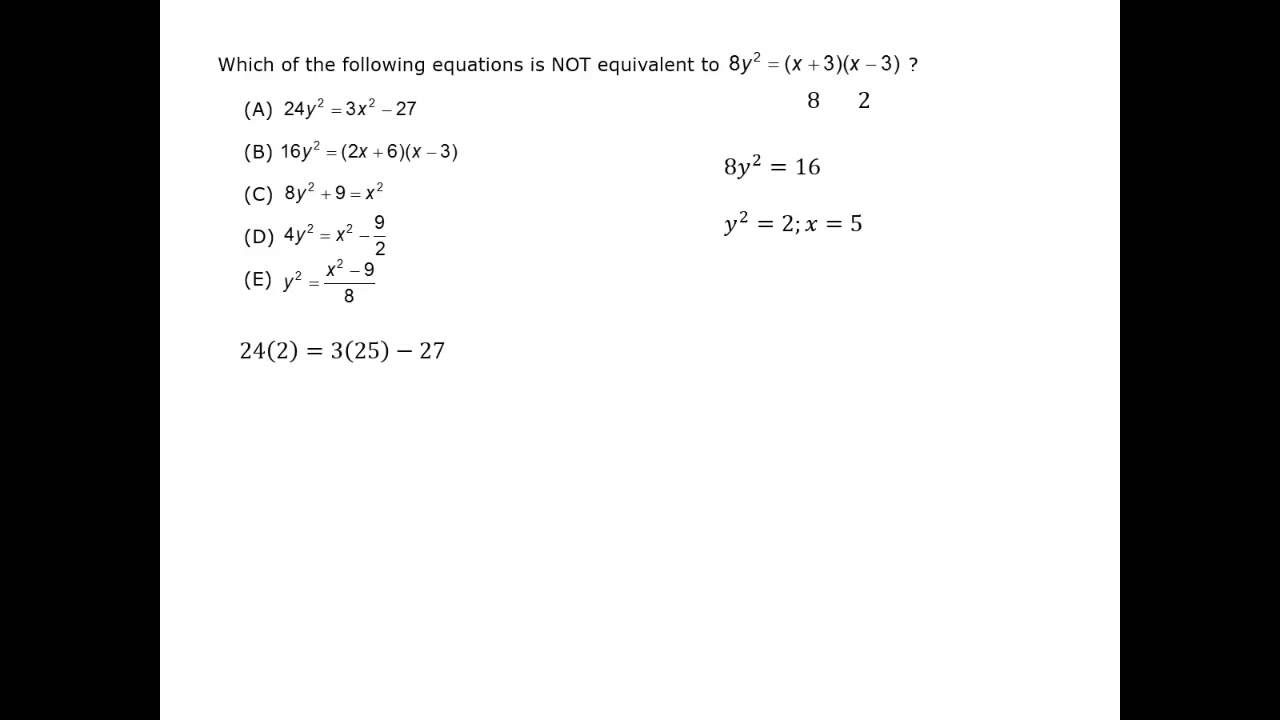## Definitions

#### Variable

A letter used to represent one or more numbers. A variable expression has numbers, variables, and operators.

#### Coefficient

The number that describes how many of the variable there are. In 4x, the coefficient is 4; there are 4 x‘s:
4x = x + x + x + x

#### Constant

is the fixed number in an equation.
In 4x + 7, the constant is 7.

#### Term

has numbers and variables, but no addition or subtraction. In the expression 4x + 7, the two terms are 4x and 7.

#### Like Terms

have the same variable and exponent. 2x with 4x, and y2 with 8y2 are pairs of like terms.

#### Expression

has addition or subtraction of terms. In the expression 4x + 7, the operation is addition.

#### Equation

has at least two expressions separated by an equals sign.
4x + 7 = 35 and y2 – 9 = 0 are equations.

#### Inequality

has at least two expressions separated by one of the symbols >, <, ≥, or ≤.

#### Linear Equation

an equation with variables and no any exponents. 4x + 7 = 35 is a linear equation but y2 – 9 = 0 is not.
The graph of a linear equation is a line. Graphing and coordinate geometry are covered in the next chapter.

#### Solution

a number or numbers that make the equation true. Equations can have one, none, or many solutions.

## Properties of Equality

Solving an equation is simplifying to get a single variable equal to a constant, such as x = 4.
When solving an equation, you need to do the same thing to both sides. View equations as a balancing act. Add, subtract, divide, multiply, square, or take the root of both sides of an equation.

Properties of equality are the specific rules for doing the same thing to both sides.

Video Courtesy of Kaplan GMAT prep.

x – 3 = 7
x – 3 + 3 = 7 + 3
x = 10

Subtraction

y + 5 = 14
y + 5 – 5 = 14 – 5
y = 9

Multiplication

\dfrac{\textit{a}}{2} = 6

2(\dfrac{\textit{a}}{2}) = 2(6)
a = 12

Division

4b = 10

\dfrac{4\textit{b}}{4} = \dfrac{10}{4}
b = 2.5

2x + 7 = 19

### Solution

2x + 7 = 19

Subtract 7 from both sides.

2x = 12

Divide both sides by 2.

x = 6

\sqrt{\textit{y}} + 1= 10

### Solution

\sqrt{\textit{y}} + 1= 10

Subtract 1 from both sides.

\sqrt{\textit{y}} = 9

Square both sides.

y = 81

2(a – 4) = 6a – 5

### Solution

2(a – 4) = 6a – 5

Use the distributive property.

2a – 8 = 6a – 5

Subtract 2a from both sides.

2a – 2a  – 8
= 6a – 2a – 5

Combine like terms. Add 5 to both sides.

-8 + 5 = 4a – 5 + 5

Combine like terms.

-3 = 4a

Divide both sides by 4.

\dfrac{-3}{4} = \textit{a}

To add or subtract polynomials, add like terms. You can use a horizontal or vertical format.

(9x3 + 2x2 + x) + (7x2– 5x + 8)

### Simplify

(9x3 + 2x2 + x) + (7x2 – 5x + 8)
= 9x3 + 2x2 + 7x2 + x – 5x + 8
= 9x3 + 9x2 – 4x + 8

(y4 + 4y3 + 2y2y – 10)
– (7y4 + 4y3 + 5y + 12)

### Simplify

xxxy4 + 4y3 + 2y2y – 10
–(7y4 + 4y3 xx+ 5y + 12)
xx-6y4 xxxxx+ 2y2 – 6y – 22

## Cross Multiplication

Cross multiplication can be used to solve rational equations. Rational equations contain fractions with variable expressions in the numerator or denominator. Multiply the numerator of each fraction by the denominator of the other fraction, and then solve the resulting equation.

\dfrac{\textit{x}}{8} = \dfrac{3}{10}

### Solution

\dfrac{\textit{x}}{8} = \dfrac{3}{10}

Cross Multiply10x = 24
x = 2.4

\dfrac{\textit{y} \,-\, 2}{3\textit{y}} = \dfrac{5}{21}

### Solution

\dfrac{\textit{y} \,-\, 2}{3\textit{y}} = \dfrac{5}{21}

Cross Multiply21(y – 2) = 15y       …Use the distributive property.
21y – 42 = 15y       …Subtract 15y and add 42 to each side.
6y = 42                    …Divide both sides by 6.
y = 7

\dfrac{\textit{a}\sqrt{2}}{8} = \dfrac{5}{4\sqrt{2}}

### Solution

\dfrac{\textit{a}\sqrt{2}}{8} = \dfrac{5}{4\sqrt{2}}

Cross Multiply(\textit{a}\sqrt{2})(4\sqrt{2}) = 5(8)        …Simplify.
4a(\sqrt{2})(\sqrt{2}) = 8a

8a = 5(8)        …Divide both sides by 8.
a = 5

\dfrac{\textit{x}}{16} = \dfrac{5}{2}

### Solution

2x = 5 × 16     …Cross multiply.

x = 40          …Divide both sides by 2.

\dfrac{\textit{x}}{\textit{x} + 4} = \dfrac{\,2\,}{3}

### Solution

3x = 2(x + 4)     …Cross multiply.

3x = 2x + 8      …Distribute the 2.

= 8             …Subtract 2x from both sides.

## Distributive Property

The distributive property is used to simplify expressions.

a(b + c) = (a × b) + (a × c) = ab + ac

2(x + 7) = 2x + (2)(7) = 2x + 14

When an equation has multiple terms, use all of the properties of equality. Apply the distributive property, then do the steps in reverse order of the order of operations: addition/subtraction, then multiplication/division, then exponents or roots.

3(x – 4)

### Simplify

3(x – 4) = 3x – (3)(4) = 3x – 12

-5(y + 2)

### Simplify

-5(y + 2) = -5y + (-5)(2) = -5y – 10.     Be sure to distribute the negative sign.

\dfrac{\textit{x}}{3} + \dfrac{\textit{x}}{2} = 5

### Solution

Multiply both sides by the least common denominator. (You can review fractions and LCD in the previous chapter.)

6(\dfrac{\textit{x}}{3} + \dfrac{\textit{x}}{2}) = 6(5)

2x + 3x = 30

5x = 30

x = 6

2a(a + 9)

### Solution

2a(a + 9) = 2a2 + 18a.            …Don’t combine 2a2 and 18a; a2 and a are not like terms.

#### Algebra Simplifying Rules

Best viewed in landscape mode

6 questions with video explanations

100 seconds per question## Multiplying Polynomials

To multiply a monomial by a monomial, multiply the numerical coefficients and each variable. (To review rules of exponents, see the Exponents section in the previous chapter.)

Multiplying polynomial expressions is based on the distributive property. To multiply a polynomial by a monomial, multiply each term of the polynomial by the monomial.

4x(3x2 – 2xy + y2)

### Simplify

#### Solution

4x(3x2 – 2xy + y2)
= 4x(3x2) – (4x)(2xy) + (4x)( y2)
= 12x3 – 8x2y + 4xy2

(2ps3)(-3p3s2)

### Simplify

(2ps3)(-3p3s2)         …Multiply coefficients:   2 × -3 = -6

= -6 × p4× s5         …and each variable:     p × p3 = p4     s3 × s2 = s5

= -6p4s5

To multiply two polynomials, multiply each term of the first expression by each term of the second. Then combine like terms.

(x + 3)(3x2 – 4x + 2)

### Simplify

(x + 3)(3x2 – 4x + 2)

x(3x2 – 4x + 2) + 3(3x2 – 4x + 2)
…Distribute x and 3 to
(3x2 – 4x + 2).

= (3x3 – 4x2 + 2x) + (9x2 – 12x + 6)      …Distribute to each term.

= 3x3 + 5x2 – 10x + 6                            …Combine like terms.

### Solving Equations with Two Variables

In some situations, you will need to solve equations with more than one variable. For example:

### Solution

The question is looking for two numbers: the number of hardcover and paperback books. Write an equation for each piece of information given in the question.

Define the variables. Let h be the number of hardcovers and p be the number of paperbacks.

She bought 8 books, so h + p = 8.

Since hardcover books cost $4, the cost of h hardcovers is 4h. Paperback books cost$2, so the cost of p paperbacks is 2p.
She spent \$28, so 4h + 2p = 28.

h + p = 8
4h + 2p = 28

Method 1: Substitution

Solve for h: h = 8 – p

Substitute:
4h + 2p = 28
4(8 – p) + 2p = 28
32 – 4p + 2p = 28
-2p = -4
p = 2, 2 paperback books

Substitute:
h + p = 8
h + 2 = 8
h = 6, 6 hardcover books

Multiply the first equation by 4:
4h + 4p = 32

Subtract:
_ [4h + 4p = 32
– [4h + 2p = 28]
2p = 4
p = 2 , 2 paperback books

Substitute:
h + p = 8
h + 2 = 8
h = 6, 6 hardcover books

#### Algebra Simplifying Rules

Best viewed in landscape mode

4 questions with video explanations

100 seconds per questionBefore attempting these problems, be sure to review this section on data sufficiency questions.

### Three or More Variables

system of linear equations consists of two or more linear equations with the same variables.  The pairs of equations solved above are linear systems.

To solve most linear systems, you need the same number of equations as variables.  For example, to find values for x and y, there were two equations.

Solving systems of equations with more than two variables is generally the same as solving for systems with two variables.  There are just more steps because there are more variables.

Solve the system of 3 linear equations:

(1) x + yz = 4
(2) x – 2y + 3z = -6
(3) 2x + 3y + z = 7

### Solution

Use the same strategy you used before. Combine pairs of equations to eliminate a variable and then substitute.

Equations (1) and (3) have +z and –z. Add the equations and the sum equation will have just two variables, x and y.

(1) 1x + 1yz = 4
(3) \underline{ 2\textit{x} + 3\textit{y} + \textit{z} = 7}
(A) 3x + 4y __= 11

Now eliminate z from a different pair of equations.

Multiply equation (1) by 3, then add equations (1) and (2).

(1) 3[x + yz = 4] → 3x + 3y – 3z = 12

(1) 3x + 3y – 3z = 12
(2) \underline{ 1\textit{x} \,-\, 2\textit{y} + 3\textit{z} = -6}
(B) 4x + 1y + 0z = 6

Combine the new equations (A) and (B) and solve for x and y.

(A) 3x + 4y = 11  and   (B) 4x + y = 6

Multiply equation (B) by 4, so y has the same coefficient.  Then subtract the equations.

(B) 4[4x + y = 6] → 16x + 4y = 24

|16x + 4y = 24
|\underline{- 3\textit{x} + 4\textit{y} = 11}       …Subtracting (A) from (B) gives a simpler equation.
|13x + 0y = 13             …So x = 1.

Substitute x = 1 into either of the equations (A) or (B).

4x + y = 6         …Substitute x = 1 into equation (B).
4(1) + y = 6      …Subtract 4 from both sides.
y = 2

Substitute the two values x = 1 and
y = 2 into any of the original equations.

x + yz = 4        …Substitute x = 1 and y = 2 into equation (1).
1 + 2 – z = 4        …Subtract 3 from both sides.
z = 1 so z = -1.

The solution for all three variables is:
x = 1, y = 2 and z = -1.

To check the answer, substitute all three values into equations (2) or (3).

(3)  2x + 3y + z = 7         …Substitute in x = 1, y = 2 and z = -1.

2(1) + 3(2) + (-1) = 7          …The answer checks.

From a bag of marbles, 15 were selected. Twice as many white marbles were selected as the number of green and blue marbles combined. One fifth of the marbles selected were green. How many marbles of each color were selected?

### Solution

The question is looking for three numbers: the number of white, green and blue marbles. Write an equation for each piece of information given in the question.

Let w be the number of white marbles, g the number of green marbles and b the number of blue marbles.

The total number of marbles is 15, so w + g + b = 15.

Twice as many white marbles as green and blue, so w = 2(g + b).
Notice that it is 2 times the green and blue, not 2 times the white.

One fifth of the total number of marbles is green, so g = (\dfrac{1}{5})(15).

The system of equations is:

(1) w + g + b = 15
(2) w = 2(g + b)
(3) g = (\dfrac{1}{5})(15)

Solving equation (3) gives g = 3.

Substitute g = 3 into equation (2).

w = 2(g + b)
w = 2(3 + b)
w = 6 + 2b

Substitute g = 3 and w = 6 + 2b into equation (1).

w + g + b = 15       …Substitute g = 3 and w = 6 + 2b.
(6 + 2b) + 3 + b = 15       …Combine like terms.
9 + 3b = 15       …Subtract 9.
3b = 6       …Divide by 3.
b = 2

So g = 3 and b = 2. Substitute into equation (2), w = 2(g + b), and w = 10.

The solution is 10 white marbles, 3 green marbles and 2 blue marbles.

## Think Before Solving

How Many Solutions?

One incorrect assumption is you can solve a linear system only if you have the same number of equations as variables. Another false assumption is that a linear system always has one answer. Systems can have one, none, or an infinite number of answers.

Is it possible to solve for x using these equations?
(1) 4x + 12y = 24
(2) \dfrac{\textit{x}}{3} + y = 2

### Solution

The quick assumption is that two equations can solve for two unknowns. The reality here is that the two statements are the same equation. Look closely: equation (1) is simply 12 times equation (2).

12(\dfrac{\textit{x}}{3} + y) = 12(2) → \dfrac{12\textit{x}}{3} + 12y
= 24 → 4x + 12y = 24

There aren’t two equations, just two versions of the same equation. This means that these two equations are not sufficient to find one solution.

This system of equations has infinitely many solutions.
For example, three possible solutions are x = 3 and y = 1; x = 0 and y = 2;
or x = -3 and y = 3.

Is it possible to solve for x using these equations?

(1) x + y = -2
(2) y = –x + 5

### Solution

Solve the first equation for y:
y = –x – 2.

Notice that the equations are now
y = –x – 2 and y = –x + 5,
saying that -2 = 5.
So even though there are two equations, there are no solutions.

**https://youtu.be/HEXbmERkDzc (hard contains more than one question))**

How Many Equations?

One incorrect assumption is that you must have the same number of equations as variables. Solving two equations for three variables can have a partial solution.

#### Shortcuts in Algebra

Remember that the GMAT is trying to measure your resourcefulness and intellect in solving problems quickly. This means that you should use shortcuts whenever you can.

Techniques such as Plugging In, Backsolving and Experimenting, which are described in the GMAT Math Strategy Introduction can be very useful. Some GMAT questions are specifically designed to be solved with these techniques, as the conventional algebra would take too much time.

Is it possible to solve for z using these equations?

(1) 2x + 4y = 12

(2) \dfrac{\textit{z}}{(\textit{x} + 2\textit{y})} = 2

### Solution

There are three variables yet only two equations.

To simplify, multiply equation (2) by
x + 2y to clear the denominator.

\dfrac{\textit{z}}{(\textit{x} + 2\textit{y})} = 2 → (x + 2y) \Big(\dfrac{\textit{z}}{\textit{x} + 2\textit{y}}\Big)
= 2(x + 2y)z = 2(x + 2y) = 2x + 4y

Now compare the simplified equations:
(2) z = 2x + 4y
(1) 2x + 4y = 12
You can see z = 12.

Notice that you can solve for z, but cannot solve further to find values for x or y. This system has an infinite number of solutions.

#### Algebra Simplifying Rules

Best viewed in landscape mode

4 questions with video explanations

100 seconds per questionBefore attempting these problems, be sure to review this section on data sufficiency questions.